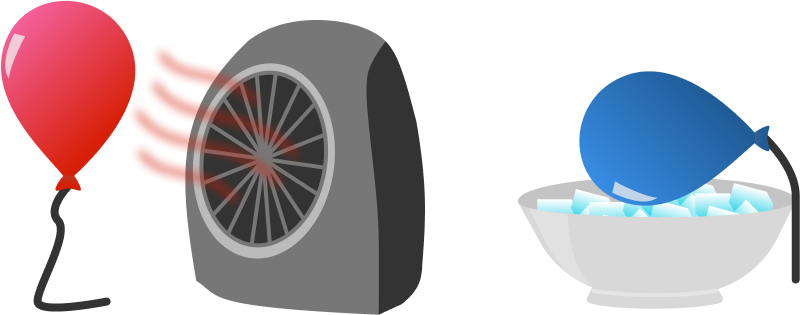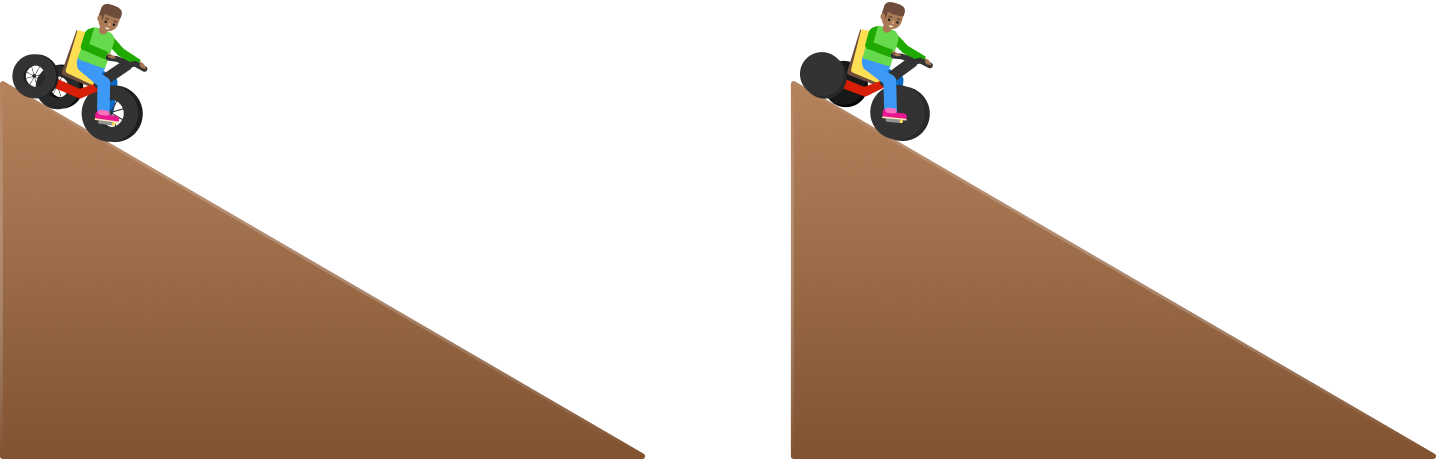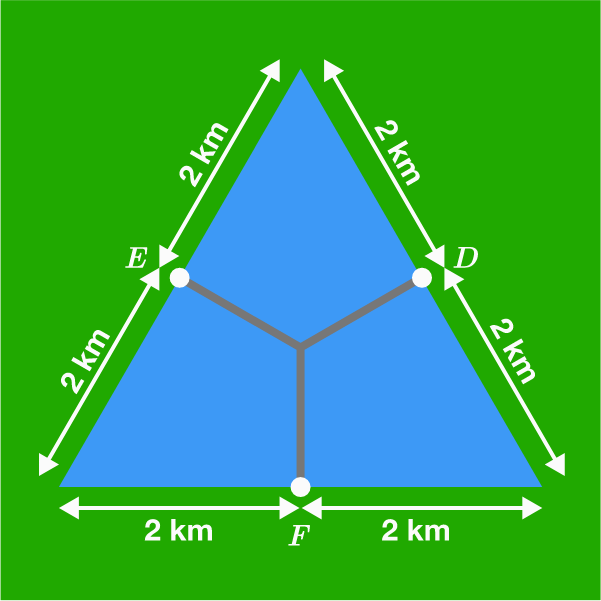# Problems of the Week

Contribute a problem

# 2018-07-23 Basic

Ram has two identical balloons filled with the same mass of helium. He heats one with a space heater and cools the other with ice, and then releases both balloons outside.

Which balloon will rise faster immediately after being released?One balloon is heated while the other is cooled.

Assumptions:

• No wind is blowing.
• The balloons don't break when heated/cooled.

$\begin{array}{rcccr} {\color{#D61F06}9^0}+9^1 & = & {\color{#D61F06}1}+9 & = & {\color{#D61F06}1}0 \\ {\color{#D61F06}9^1}+9^2 & = & {\color{#D61F06}9}+81 & = & {\color{#D61F06}9}0 \\ {\color{#D61F06}9^2}+9^3 & = & {\color{#D61F06}81}+729 & = & {\color{#D61F06}81}0 \\ \end{array}$

Is the following true for any positive integer $n?$ ${\color{#D61F06}9^n}+9^{n+1}={\color{#D61F06}9^n} \times 10$On the left, a cyclist rolls down a hill without pedaling and without using his brakes. On the right, he does the same but has replaced his wheels with disks.

Which way is faster?

Details and Assumptions:

• The disks are uniform and have the same mass and radius as the wheels.
• The friction is sufficient to avoid slipping of the wheels.
• Neglect the mass of the spokes.

Architect Bob has designed a Y-shaped bridge across Lake Delta (in blue) to connect towns $D, E,$ and $F.$ The three arms of the bridge intersect at the centroid of the triangle.

What is the total length of Bob's bridge in kilometers?We know $2 + 2 = 2\times 2.$

But are there two distinct positive integers $a$ and $b$ such that their sum and their product are equal: $a + b = a\times b ?$

×